WOD/Pre-patch - Page 13 - WoWInterface

11-09-14, 04:45 PM   #241
castersrus
A Fallenroot Satyr
Join Date: Dec 2010
Posts: 24
 Originally Posted by Rythal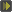It's not on curse or WoWI yet, I've been utilizing github so people can help out with getting parts changed to the new API and fixing bugs that crop up, so when I say I pushed an update it means I sync'd what is on github with my current version.
Ahhhhh, got ya. Looking forward to the release of full version...can't wait. Like everyone else this is one of the mods that I'm missing the function of most. Thank you for all the time you put into this
__________________
Peel away my reality and reveal the insanity within

11-09-14, 05:10 PM   #242
Svegan
A Warpwood Thunder Caller
Join Date: Jun 2010
Posts: 99
Instances

Instances seem to work fine for me. I think it interesting though, that they show as scenario in the carbonite quest watch window. could that be due to the bonus objective feature now, for killing all dungeon bosses?

11-09-14, 05:23 PM   #243
Naharis
A Defias Bandit
Join Date: Aug 2012
Posts: 3
I created a pull request for my changes on WatchList items, although I feel it's far from finished.
Just did some testing with it, there's still a problem when finishing an item quest during combat lockdown. My list updated and removed the button after leaving combat, but it duplicated another button on the list.

11-09-14, 06:29 PM   #244
Tokyn
A Kobold Labourer
Join Date: Nov 2014
Posts: 1
Guys,

Thanks for the hard work you are putting in. I see that people are starting to use the Alpha version and post some feed back. I hope that everyone doesn't over do it and post all their issues. As we all know this is a work in process so most of the errors we are getting will work out with the major updates.

Once again thanks for bringing back some of the function of Carb II, I actually stopped playing until today because of the mod being down.

You all rock!

11-09-14, 06:59 PM   #245
crzyface
A Deviate Faerie Dragon
Join Date: Oct 2007
Posts: 18
10 man blackwing descent error happened when i entered and opened map to access instance map.
while running the instance i noticed the error got triggered by mouseover

Code:
```Message: Interface\AddOns\Carbonite\NxMap.lua:9025: Usage: GetMapNameByID(mapID)
Time: 11/09/14 18:56:11
Count: 3589
Stack: [C]: in function `GetMapNameByID'
Interface\AddOns\Carbonite\NxMap.lua:9025: in function `IdToName'
Interface\AddOns\Carbonite\NxMap.lua:5813: in function `CheckWorldHotspots'
Interface\AddOns\Carbonite\NxMap.lua:3810: in function <Interface\AddOns\Carbonite\NxMap.lua:3673>

Locals: (*temporary) = nil```

Last edited by crzyface : 11-09-14 at 07:40 PM. Reason: update

11-09-14, 11:05 PM   #246
Svegan
A Warpwood Thunder Caller
Join Date: Jun 2010
Posts: 99
Social and Punks

The error message I posted earlier, is related to Social and Punks module. I can turn it off, leave all the others running, and I HAVE maps and warehouse, and questing. I am so happy.

11-09-14, 11:21 PM   #247
Thrumbar
A Chromatic Dragonspawn
Join Date: Jan 2005
Posts: 156Getting these errors as of Sunday Night after last push

Lua Code:
`5185x Carbonite\NxMap.lua:9677: attempt to concatenate local 'tip' (a nil value)Carbonite\NxMap.lua:9677: in function `MapAddIcon'Wholly\Wholly-046.lua:3373: in function `ShowPin'Wholly\Wholly-046.lua:3704: in function `_UpdatePins'Wholly\Wholly-046.lua:1068: in function `f'Grail\Grail-066PR1.lua:5224: in function `_PostNotification'Grail\Grail-066PR1.lua:5405: in function `_ProcessDelayedNotifications'Grail\Grail-066PR1.lua:5213: in function <Grail\Grail.lua:5213> Locals:self = <table> { SortingFunction = <function> defined @Wholly\Wholly.lua:3482 pinsNeedFiltering = false _WorldMapUpdateHandler = <function> defined @Wholly\Wholly.lua:1058 configurationScript5 = <function> defined @Wholly\Wholly.lua:399 _BreadcrumbQuestId = <function> defined @Wholly\Wholly.lua:969 Sort = <function> defined @Wholly\Wholly.lua:3453 _SearchFrameShow = <function> defined @Wholly\Wholly.lua:3019 pins = <table> { } _RemoveAllDirectionalArrows = <function> defined @Wholly\Wholly.lua:2942 currentMaximumTooltipLines = 50 debug = true configurationScript2 = <function> defined @Wholly\Wholly.lua:384 tooltip = com_mithrandir_WhollyTooltip { } SortButtonEnter = <function> defined @Wholly\Wholly.lua:3467 initialUpdateProcessed = false dropdownText = "Kun-Lai Summit  " ScrollFrame_Update_WithCombatCheck = <function> defined @Wholly\Wholly.lua:2998 levelTwoData = <table> { } _ColorCodeFromInfo = <function> defined @Wholly\Wholly.lua:1173 ScrollFrame_Update = <function> defined @Wholly\Wholly.lua:3110 cachedPanelQuests = <table> { } levelTwoCurrent = <table> { } configurationScript10 = <function> defined @Wholly\Wholly.lua:412 carboniteMapLoaded = true playerAliveReceived = false _HideTooltip = <function> defined @Wholly\Wholly.lua:1670 QuestInfoEnter = <function> defined @Wholly\Wholly.lua:2795 configurationScript12 = <function> defined @Wholly\Wholly.lua:419 SetCurrentZoneToPanel = <function> defined @Wholly\Wholly.lua:3284 _NPCInfoSection = <function> defined @Wholly\Wholly.lua:2019 carboniteRemoveWaypointFunction = <function> defined @Carbonite\NxMap.lua:9624 receivedCalendarUpdateEventList = false _RecordTooltipNPCs = <function> defined @Wholly\Wholly.lua:2888 ShowBreadcrumbInfo = <function> defined @Wholly\Wholly.lua:3312 _Dropdown_GetText = <function> defined @Wholly\Wholly.lua:1387 waypoints = <table> { } configurationScript8 = <function> defined @Wholly\Wholly.lua:405 _DroppedItemMatchesQuest = <function> defined @Wholly\Wholly.lua:1430 _TagDeleteConfirm = <function> defined @Wholly\Wholly.lua:3543 checkedNPCs = <table> { } color = <table> { } _OnEvent = <function> defined @Wholly\Wholly.lua:2097 _SetLevelTwoCurrent = <function> defined @Wholly\Wholly.lua:3300 _QuestInfoTurninSection = <function> defined @Wholly\Wholly.lua:2848 npcs = <table> { } _Dropdown_Create = <function> defined @Wholly\Wholly.lua:1376 carboniteNxMapOpen = <function> defined @Carbonite\NxMap.lua:206 Click = <function> defined @Wholly\Wholly.lua:1136 playerClassMap = 200015 preferenceButtons = <table> { } _DisplayMapFrame = <function> defined @Wholly\Wholly.lua:1347 supportedControlMaps = <table> { } _ColorUpdatePreferenceText = <function> defined @Wholly\Wholly.lua:1202 ToggleSwitch = <function> defined @Wholly\Wholly.lua:3614 Dropdown_Initialize = <function> defined @Wholly\Wholly.lua:1394 filteredPinQuests = <table> { } ScrollFrame_OnLoad = <function> defined @Wholly\Wholly.lua:2980 filteredPanelQuests = <table> { } BreadcrumbClick = <function> defined @Wholly\Wholly.lua:948 BreadcrumbUpdate = <function> defined @Wholly\Wholly.lua:982 _PrettyQuestString = <function> defined @Wholly\Wholly.lua:2386 pairedConfigurationButton = com_mithrandir_whollyConfigFrameButton21 { } _Dropdown_AddButton = <function> defined @Wholly\Wholly.lua:1356 UpdateBreadcrumb = <function> defined @Wholl`

Lua Code:
`38x Carbonite\Carbonite-6.0.lua:3815: bad argument #1 to 'find' (string expected, got nil)[C]: in function `find'Carbonite\Carbonite-6.0.lua:3815: in function `Split'Carbonite.Quests\NxQuest.lua:9661: in function `Unpack'Carbonite.Quests\NxQuest.lua:4416: in function `CheckShow'Carbonite.Quests\NxQuest.lua:10026: in function `CalcDistances'Carbonite.Quests\NxQuest.lua:8426: in function `func'DataStore\libs\AceTimer-3.0\AceTimer-3.0-17.lua:55: in function <DataStore\libs\AceTimer-3.0\AceTimer-3.0.lua:48> Locals:d = "|"p = nilTempNum = 0Tossaway = <table> {}TempTable = <table> { I^141558549800^0^894d58^+13150 xp = <table> { } SPELL_ABSORBED = <table> { } I^141558816301^0^72be04^+424 xp = <table> { } 1|1|1|.6 = <table> { } |807|32|73.685765|85.216534 = <table> { } I^141558554300^0^8a7d4d^+13150 xp = <table> { } I^141558857101^0^6c0dbf^+424 xp = <table> { } I^141558954101^0^b94bb7^+128 xp = <table> { } K^141558966500^0^a17655^Needle Sprite^31 = <table> { } I^141558839601^0^6dedfd^+424 xp = <table> { } I^141558842001^0^6d5df9^+424 xp = <table> { } I^141558808201^0^71ee2c^+2 xp = <table> { } K^141558945100^0^b89b7d^Needle Sprite^4 = <table> { } I^141558861401^0^6d9dc0^+424 xp = <table> { } K^141558950300^0^ba1b99^Needle Sprite^12 = <table> { } K^141558961600^0^58c8cc^Needle Sprite^27 = <table> { } [[Beyond the Wall|2|88||]] = <table> { } K^141558958100^0^2d8579^Needle Sprite^22 = <table> { } I^141558958202^0^2d8579^+128 xp = <table> { } K^141558956800^0^2fb543^Needle Sprite^20 = <table> { } K^141558945600^0^b8ab7c^Needle Sprite^6 = <table> { } K^141558969000^0^a64959^Needle Sprite^34 = <table> { } I^141558821300^0^724e2a^+4 xp = <table> { } I^141558956801^0^2fb543^+128 xp = <table> { } Repel Boarders!|1|30|28|0|0 = <table> { } SPELL_AURA_REMOVED = <table> { } I^141558970101^0^a5598a^+128 xp = <table> { } I^141558943601^0^b76b7c^+128 xp = <table> { } I^141558939400^0^b7fb3a^+1300 xp = <table> { } I^141558963201^0^6e65c2^+128 xp = <table> { } I^141558970401^0^a5598a^+128 xp = <table> { } I^141558951701^0^ba8bac^+128 xp = <table> { } |809|32|67.854857|73.995006|6|6 = <table> { } SPELL_AURA_APPLIED = <table> { } I^141558950201^0^ba1b99^+128 xp = <table> { } K^141558958201^0^2d8579^Needle Sprite^24 = <table> { } K^141558857100^0^6c0dbf^Blind Rage^10 = <table> { } K^141558960700^0^58c8cc^Needle Sprite^25 = <table> { } |807|32|53.583860|51.242018 = <table> { } I^141558813600^0^725e2a^+4 xp = <table> { } K^141558837600^0^6eedf9^Blind Rage^2 = <table> { } 0|0|0|.313 = <table> { } I^141558939500^0^b7fb3a^+13300 xp = <table> { } I^141558971500^0^a3d9df^+13300 xp = <table> { } SPELL_ENERGIZE = <table> { } [[Everything I Know About Cooking|2|90||]] = <table> { } SPELL_PERIODIC_DAMAGE = <table> { } K^141558863200^0^6f3dcf^Blind Rage^12 = <table> { } UNIT_DIED = <table> { } I^141558821900^0^728e21^+4 xp = <table> { } I^141558956601^0^2ef533^+128 xp = <table> { } K^141558949600^0^ba1b99^Needle Sprite^10 = <table> { } I^141558953801^0^b94bb5^+128 xp = <table> { } I^141558935901^0^b31b24^+424 xp = <table> { } [[Muskpaw Ranch|2|87||]] = <table> { } K^141558813100^0^725e2a^Sha-Infested Yaungol^3 = <table> { } I^141558947201^0^b9eb81^+128 xp = <table> { } I^141558945601^0^b8ab7c^+128 xp = <table> { } K^141558840300^0^6dddfc^Blind Rage^4 = <table> { } K^141558852500^0^698dd3^Blind Rage^8 = <table> { } K^141558816300^0^72be04^Sha-Infested Yaungol^4 = <table> { } SPELL_MISSED = <table> { } I^141558949601^0^ba1b99^+128 xp = <table> { } I^141558881800^0^71ae5f^+13150 xp = <table> { } SPELL_CAST_START = <table> { } I^141558836501^0^6eedfb^+424 xp = <table> { } K^141558963000^0^6e65c2^Needle Sprite^28 = <table> { } I^141558943401^0^b76b7c^+128 xp = <table> { } I^141558787500^0^71be5e^+1300 xp = <table> { } 2114|607|32|71.04|49.94 = <table> { } [[The Fearmaster|2|88||]] = <table> { } I^141558950301^0^ba1b99^+128 xp = <table> { } K^141558943400^0^b76b7c^Needle Sprite^2 = <table> { } I^141558896101^0^726dc7^+424 xp = <table> { } |809|32|12.788630|26.837820|6|6 = <table> { } K^141558845000^0^6b7dfc^Blind Rage^6 = <table> { } [[The Late Mrs. Muskpaw|2|88||]] = <table> { } K^141558961300^0^58c8cc^Needle Sprite^26 = <table> { } K^141558504200^0^7eac53^Ordo Battleyak^1 = <table> { } K^141558510700^0^7c2c41^Summoned Searing Totem^1 = <table> { } K^141558957200^0^2d8579^Needle Sprite^21 = <table> { } K^141558970400^0^a5598a^Needle Sprite^37 = <table> { } SPELL_CAST_FAILED = <table> { } |809|32|4`

Lua Code:
`14x Carbonite.Quests\NxQuest.lua:7584: attempt to index field '?' (a nil value)Carbonite.Quests\NxQuest.lua:7584: in function `func'DataStore\libs\AceTimer-3.0\AceTimer-3.0-17.lua:55: in function <DataStore\libs\AceTimer-3.0\AceTimer-3.0.lua:48> Locals:timer = <table> { 1 = <table> { } argsCount = 1 object = <table> { } ends = 21184.609 callback = <function> defined @DataStore\libs\AceTimer-3.0\AceTimer-3.0.lua:48 func = <function> defined @Carbonite.Quests\NxQuest.lua:7557 delay = 0.01}type = <function> defined =[C]:-1unpack = <function> defined =[C]:-1GetTime = <function> defined =[C]:-1C_TimerAfter = <function> defined =[C]:-1activeTimers = <table> { (null) = <table> { } (null) = <table> { } (null) = <table> { } (null) = <table> { } (null) = <table> { } (null) = <table> { } (null) = <table> { } (null) = <table> { } (null) = <table> { } (null) = <table> { } (null) = <table> { } (null) = <table> { } (null) = <table> { } (null) = <table> { } (null) = <table> { } (null) = <table> { } (null) = <table> { } (null) = <table> { } (null) = <table> { } (null) = <table> { } (null) = <table> { } (null) = <table> { } (null) = <table> { } (null) = <table> { } (null) = <table> { } (null) = <table> { } (null) = <table> { } (null) = <table> { } (null) = <table> { } (null) = <table> { } (null) = <table> { } (null) = <table> { } (null) = <table> { } (null) = <table> { } (null) = <table> { } (null) = <table> { } (null) = <table> { } (null) = <table> { } (null) = <table> { } (null) = <table> { } (null) = <table> { } (null) = <table> { } (null) = <table> { } (null) = <table> { } (null) = <table> { } (null) = <table> { } (null) = <table> { } (null) = <table> { } (null) = <table> { } (null) = <table> { } (null) = <table> { } (null) = <table> { } (null) = <table> { } (null) = <table> { } (null) = <table> { } (null) = <table> { } (null) = <table> { } (null) = <table> { } (null) = <table> { } (null) = <table> { } (null) = <table> { } (null) = <table> { } (null) = <table> { } (null) = <table> { } (null) = <table> { } (null) = <table> { } (null) = <table> { } (null) = <table> { } (null) = <table> { } (null) = <table> { } (null) = <table> { } (null) = <table> { } (null) = <table> { } (null) = <table> { } (null) = <table> { } (null) = <table> { } (null) = <table> { } (null) = <table> { } (null) = <table> { } (null) = <table> { } (null) = <table> { } (null) = <table> { } (null) = <table> { } (null) = <table> { } (null) = <table> { } (null) = <table> { } (null) = <table> { } (null) = <table> { } (null) = <table> { } (null) = <table> { } (null) = <table> { } (null) = <table> { } (null) = <table> { } (null) = <table> { } (null) = <table> { } (null) = <table> { } (null) = <table> { } (null) = <table> { } (null) = <table> { } (null) = <table> { } (null) = <table> { } (null) = <table> { } (null) = <table> { } (null) = <table> { } (null) = <table> { } (null) = <table> { } (null) = <table> { } (null) = <table> { } (null) = <table> { } (null) = <table> { } (null) = <table> { } (null) = <table> { } (null) = <table> { } (null) = <table> { } (null) = <table> { } (null) = <table> { } (null) = <table> { } (null) = <table> { } (null) = <table> { } (null) = <table> { } (null) = <table> { } (null) = <table> { } (null) = <table> { } (null) = <table> { } (null) = <table> { } (null) = <table> { } (null) = <table> { } (null) = <table> { } (null) = <table> { } (null) = <table> { } (null) = <table> { } (null) = <table> { } (null) = <table> { } (null) = <table> { } (null) = <table> { } (null) = <table> { } (null) = <table> { } (null) = <table> { } (null) = <table> { } (null) = <table> { } (null) = <table> { } (null) = <table> { } (null) = <table> { } (null) = <table> { } (null) = <table> { } (null) = <table> { } (null) = <table> { } (null) = <table> { } (null) = <table> { } (null) = <table> { } (null) = <table> { } (null) = <table> { } (null) = <table> { } (null) = <table> { } (null) = <table> { } (null) = <table> { } (null) = <table> { } (null) = <table> { } (n`

Lua Code:
`3397x Carbonite\NxUI.lua:4941: CreateFrame(): Couldn't find inherited node "WatchFrameItemButtonTemplate"[C]: in function `CreateFrame'Carbonite\NxUI.lua:4941: in function `GetFrame'Carbonite\NxUI.lua:5673: in function `Update'Carbonite.Quests\NxQuest.lua:8928: in function `UpdateList'Carbonite.Quests\NxQuest.lua:8436: in function `func'DataStore\libs\AceTimer-3.0\AceTimer-3.0-17.lua:55: in function <DataStore\libs\AceTimer-3.0\AceTimer-3.0.lua:48> Locals:self = <table> { ItemSetFunc = <function> defined @Carbonite\NxUI.lua:6050 OpenColorDialog = <function> defined @Carbonite\NxUI.lua:6294 ItemSetDataEx = <function> defined @Carbonite\NxUI.lua:6128 SetFade = <function> defined @Carbonite\NxUI.lua:5126 ClickToName = <table> { } ItemGetNum = <function> defined @Carbonite\NxUI.lua:5979 SendUserSelect = <function> defined @Carbonite\NxUI.lua:6162 ItemSetColorButton = <function> defined @Carbonite\NxUI.lua:6067 Sort = <function> defined @Carbonite\NxUI.lua:5893 ItemSetButtonTip = <function> defined @Carbonite\NxUI.lua:6038 SetUser = <function> defined @Carbonite\NxUI.lua:5117 Empty = <function> defined @Carbonite\NxUI.lua:5843 Resize = <function> defined @Carbonite\NxUI.lua:5262 ItemAdd = <function> defined @Carbonite\NxUI.lua:5973 GetSize = <function> defined @Carbonite\NxUI.lua:5254 CreateStrings = <function> defined @Carbonite\NxUI.lua:5367 SetItemFrameScaleAlpha = <function> defined @Carbonite\NxUI.lua:5200 Select = <function> defined @Carbonite\NxUI.lua:6137 SetBGColor = <function> defined @Carbonite\NxUI.lua:5151 Create = <function> defined @Carbonite\NxUI.lua:4994 ItemGetData = <function> defined @Carbonite\NxUI.lua:6104 Lists = <table> { } SaveColumns = <function> defined @Carbonite\NxUI.lua:5746 GetFrame = <function> defined @Carbonite\NxUI.lua:4927 FrmsUniqueI = 1 Frms = <table> { } SaveData = <table> { } OnSlider = <function> defined @Carbonite\NxUI.lua:6344 OnBut = <function> defined @Carbonite\NxUI.lua:6278 ColumnAdd = <function> defined @Carbonite\NxUI.lua:5762 ItemGetFunc = <function> defined @Carbonite\NxUI.lua:6057 GetSelected = <function> defined @Carbonite\NxUI.lua:6154 OnHdrMouseDown = <function> defined @Carbonite\NxUI.lua:6188 CBaseLineH = 12 OnMouseDown = <function> defined @Carbonite\NxUI.lua:6230 Update = <function> defined @Carbonite\NxUI.lua:5477 ItemSetOffset = <function> defined @Carbonite\NxUI.lua:6094 SetMinSize = <function> defined @Carbonite\NxUI.lua:5226 ItemGetDataEx = <function> defined @Carbonite\NxUI.lua:6119 IsShowLast = <function> defined @Carbonite\NxUI.lua:6181 CreateButtons = <function> defined @Carbonite\NxUI.lua:5432 SetSize = <function> defined @Carbonite\NxUI.lua:5235 ShowLast = <function> defined @Carbonite\NxUI.lua:6172 __index = <table> { } Lock = <function> defined @Carbonite\NxUI.lua:5208 ColumnHitTest = <function> defined @Carbonite\NxUI.lua:5826 ItemSetFrame = <function> defined @Carbonite\NxUI.lua:6084 OnMouseWheel = <function> defined @Carbonite\NxUI.lua:6259 Init = <function> defined @Carbonite\NxUI.lua:4859 ItemGetButtonTip = <function> defined @Carbonite\NxUI.lua:6031 FreeFrames = <function> defined @Carbonite\NxUI.lua:4896 ItemSetButton = <function> defined @Carbonite\NxUI.lua:6009 ItemSet = <function> defined @Carbonite\NxUI.lua:5984 ColumnSetName = <function> defined @Carbonite\NxUI.lua:5804 ColumnSort = <function> defined @Carbonite\NxUI.lua:5875 GetLineH = <function> defined @Carbonite\NxUI.lua:5193 SetCreateFont = <function> defined @Carbonite\NxUI.lua:4975 SetLineHeight = <function> defined @Carbonite\NxUI.lua:5180 FullUpdate = <function> defined @Carbonite\NxUI.lua:5217 NextUpdat`

The last on I think relates to quest use items that appear in quest tracker nest to quest.

 11-10-14, 01:20 AM #248 Rythal Featured Artist Join Date: Aug 2012 Posts: 1,458 Is anyone else finding super lag while Questing addon is enabled? I already know what function call is causing it, but I'm surprised it hasn't been commented on so I'm wondering if it's just me.
11-10-14, 01:53 AM   #249
Boggtroll
A Deviate Faerie Dragon
Join Date: Oct 2014
Posts: 12
do you just download the file on the git and put them in a folder? if so i would be willing to test as extra input.

11-10-14, 02:43 AM   #250
ircdirk
A Molten Giant
Join Date: Nov 2014
Posts: 817
 Originally Posted by Boggtrolldo you just download the file on the git and put them in a folder? if so i would be willing to test as extra input.
Just use the "Download ZIP" button on right on GitHub, extract to addons folder (first delete old carbonite addons) and thats all.

Last edited by ircdirk : 11-10-14 at 02:45 AM.

11-10-14, 03:10 AM   #251
samyonair
A Frostmaul Preserver
Join Date: May 2009
Posts: 257
Missing Flightmasters - locales files?

Hi Rythal,

in Pandaria, these flightmasters are not in your locales files, i have added them in the german flightmasters.lua , but d'ont know if they are missing in other files to.
Code:
```L["Beeble's Wreck, Isle Of Giants"] = "Beebles Wrack, Insel der Riesen"
L["Bozzle's Wreck, Isle Of Giants"] = "Bozzles Wrack, Insel der Riesen"
L["Huojin Landing, Timeless Isle"] = "Landungstelle der Huojin, Zeitlose Insel"
L["Tushui Landing, Timeless Isle"] = "Landungstelle der Tushui, Zeitlose Insel"```
i've insert them on the lines in alphabetical order

greetings Samyonair

11-10-14, 04:29 AM   #252
dvantassel11
A Cyclonian
Join Date: Apr 2012
Posts: 46
PetTracker

I get this error in PetTracker when Carb Quests is loaded using the current Git version

Date: 2014-11-10 05:26:35
ID: 1
Error occured in: Global
Count: 1
Message: ..\AddOns\PetTracker_Config\Tutorial.lua line 136:
attempt to index field 'Objectives' (a nil value)
Debug:
PetTracker_Config\Tutorial.lua:136: in main chunk
[C]: LoadAddOn()
...nterface\AddOns\PetTracker\Features\ConfigLoader.lua:23: ?()
PetTracker\PetTracker.lua:40: ForAllModules()
PetTracker\PetTracker.lua:54: ?()
PetTracker\PetTracker.lua:21:
PetTracker\PetTracker.lua:21
Locals:
Addon = <table> {
Switcher = PetTrackerSwap {
}
GetTypeIcon = <function> defined @Interface\AddOns\PetTracker\Core\Static.lua:102
Tamer = <table> {
}
PLAYER_ENTERING_WORLD = <function> defined @Interface\AddOns\PetTracker\PetTracker.lua:48
ForAllModules = <function> defined @Interface\AddOns\PetTracker\PetTracker.lua:37
MaxLevel = 25
TamerPet = <table> {
}
Species = <table> {
}
JournalSlot = <table> {
}
Tutorial = <table> {
}
State = <table> {
}
ConfigLoader = <table> {
}
BattleSlot = <table> {
}
GetQualityColor = <function> defined @Interface\AddOns\PetTracker\Core\Static.lua:90
Sets = <table> {
}
Journal = <table> {
}
Line = <table> {
}
StableBlip = <table> {
}
WorldMap = PetTrackerMapFilter {
}
EnemyActions = PetTrackerEnemyActions {
}
Filter = <function> defined @Interface\AddOns\PetTracker\Core\Filters.lua:23
Locals = <table> {
}
Tracker = <table> {
}
MapTip = <table> {
}
List = <table> {
}
NewClass = <function> defined @Interface\AddOns\PetTracker\PetTracker.lua:27
Stats = <table> {
}
SourceIcons = <table> {
}
BattleListener = <unnamed> {
}
MaxQuality = 4
WORLD_MAP_UPDATE = <function> defined @Interface\AddOns\PetTracker\PetTracker.lua:62
ProgressBar = <table> {
}
GetDate = <function> defined @Interface\AddOns\PetTracker\Core\Static.lua:134
GetTypeName = <function> defined @Interface\AddOns\PetTracker\Core\Static.lua:98
Battle = <table> {
}
Blip = <table> {
}
BreedStats = <table> {
}
TamerLandmarks = <table> {
}
PET_JOURNAL_LIST_UPDATE = <function> defined @Interface\AddOns\PetTracker\PetTracker.lua:75
UnpackDate = <function> defined @Interface\AddOns\PetTracker\Core\Static.lua:126
Tamers = <table> {
}
Stables = <table> {
}
TamerOrder = <table> {
}
AbilityAction = <table> {
}
QualityScale = <table> {
}
Specie = <table> {
}
BreedNames = <table> {
}
ContinentByZone = <table> {
}
Breeds = <table> {
}
AbilityButton = <table> {
}
KeepShort = <function> defined @Interface\AddOns\PetTracker\Core\Static.lua:118
Predict = <table> {
}
GetBreedIcon = <function> defined @Interface\AddOns\PetTracker\Core\Static.lua:110
GetBreedName = <function> defined @Interface\AddOns\PetTracker\Core\Static.lua:106
BreedIcons = <table> {
}
SpecieBlip = <table> {
}
NewModule = <function> defined @Interface\AddOns\PetTracker\PetTracker.lua:31
}
ADDON = "PetTracker"
Tutorial = <table> {
Reset = <function> defined @Interface\AddOns\PetTracker_Config\Tutorial.lua:99
Trigger = <function> defined @Interface\AddOns\PetTracker_Config\Tutorial.lua:95
Split = <function> defined @Interface\AddOns\PetTracker_Config\Tutorial.lua:34
Start = <function> defined @Interface\AddOns\PetTracker_Config\Tutorial.lua:26
Register = <function> defined @Interface\AddOns\PetTracker_Config\Tutorial.lua:87
Restart = <function> defined @Interface\AddOns\PetTracker_Config\Tutorial.lua:78
HookJournal = <function> defined @Interface\AddOns\PetTracker_Config\Tutorial.lua:52
}
Lib = <table> {
RegisterTutorials = <function> defined @Interface\AddOns\PetTracker\Libs\CustomTutorials-2.1\CustomTutorials-2.1.lua:172
numFrames = 1
GetTutorials = <function> defined @Interface\AddOns\PetTracker\Libs\CustomTutorials-2.1\CustomTutorials-2.1.lua:213
ResetTutorials = <function> defined @Interface\AddOns\PetTracker\Libs\CustomTutorials-2.1\CustomTutorials-2.1.lua:198
TriggerTutorial = <function> defined @Interface\AddOns\PetTracker\Libs\CustomTutorials-2.1\CustomTutorials-2.1.lua:181
frames = <table> {
}
}
L = <table> {
UnlockActions = "Unlock Enemy Actions"
Tutorial = <table> {
}
TellMore = "Tell me more about yourself."
AskForfeit = "No upgrades are available. Quit battle?"
Maximized = "Maximized"
JournalTutorial = <table> {
}
Ninja = "Ninja"
AddWaypoint = "Add Waypoint"
AvailableBreeds = "
Available Breeds:"
ShowStables = "Show Stables"
Breed = "Breed"
AlertUpgrades = "Alert for Upgrades"
EnemyTeam = "Enemy Team"
Source4 = "Profession"
Source6 = "Achievement"
ShowJournal = "Show in Journal"
UpgradeAlert = "Wild upgrades have appeared!"
Source10

11-10-14, 07:47 AM   #253
Thrumbar
A Chromatic Dragonspawn
Join Date: Jan 2005
Posts: 156
 Originally Posted by RythalIs anyone else finding super lag while Questing addon is enabled? I already know what function call is causing it, but I'm surprised it hasn't been commented on so I'm wondering if it's just me.
Nope, I have it to. Makes It difficult to play on a pvp server..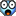11-10-14, 07:54 AM   #254
kermit
A Defias Bandit
Join Date: Nov 2014
Posts: 2
Hi All

First off like to say keep up the hard work.

I'm have issue with the alpha , i got the update off curse and and have installed it. My map is still showing the blizzard map but with a C on it. if i left click on the C nothing happens and if i hit M for map nothing opens even the old one doesnt.

11-10-14, 08:30 AM   #255
ircdirk
A Molten Giant
Join Date: Nov 2014
Posts: 817
 Originally Posted by kermitHi All First off like to say keep up the hard work. I'm have issue with the alpha , i got the update off curse and and have installed it. My map is still showing the blizzard map but with a C on it. if i left click on the C nothing happens and if i hit M for map nothing opens even the old one doesnt.
Dont use curse version for 6.0.3... its outdated and not for this WoW version. U have to download it from GitHub: https://github.com/Rythal/Carbonite buts its like pre alpha version for now.

11-10-14, 09:10 AM   #256
kermit
A Defias Bandit
Join Date: Nov 2014
Posts: 2
That did it thanks alot for that info , keep up the good work

maps are all good

 11-10-14, 09:43 AM #257 Rythal Featured Artist Join Date: Aug 2012 Posts: 1,458 Trying to get the Draenor stuff added, but i'll be damned if I can remember the formula to calculate the numbers based on the info inside the dbc file.. I have the map scale, and I have the Y, but I can't remember the X.
11-10-14, 10:28 AM   #258
atl77
A Chromatic Dragonspawn
Join Date: Oct 2014
Posts: 179
German translation

I have forked the repository and started on a german translation: https://github.com/atk/Carbonite - I'll probably be finished in 2-3 days.

11-10-14, 11:18 AM   #259
JimJoBlue
An Onyxian Warder
Join Date: Aug 2012
Posts: 362
 Originally Posted by atl77I have forked the repository and started on a german translation: https://github.com/atk/Carbonite - I'll probably be finished in 2-3 days.
You might want to coordinate your efforts with Samyonair in this thread.. He/She is already working on a deDE conversion... Perhaps you could work together?
__________________
I still say a church steeple with a lightning rod on top shows a lack of confidence. Doug McLeod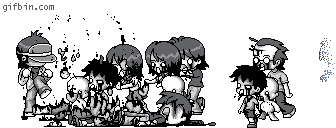11-10-14, 11:48 AM   #260
Draven006
A Fallenroot Satyr
Join Date: Sep 2012
Posts: 23
Ok guys this is a strange one

Not that it need to be fixed right now as i know how much Rythal is doing to get this awesome addon working again
But here goes.

IF you have Archaeology as a profession, AND you use the addon Archy, when you go to the world map you cannot view the map of other zones.

IF you have Archaeology as a profession, AND you DONT use the addon Archy, the the map works like a charm.

IF you DONT have Archaeology as a profession, AND you still have the addon Archy activated then the world map works like a charm.

So the conclusion is that WITH THE PROFESSION and the ARCHY addon, something must be getting written to the map (like where the digsites are or something) that stops the other zones in the map from being viewed.

A long time ago i discovered this and spoke to the Developer of Archy and he called out that it was carbonites fault NOT his, this was of corse back in the day when Carbonite was a paid for addon and THOSE Developers were not prepared to do anything about it (even though i was a paying customer)

So maybe while the code is being looked at, i was wondering if there is anything that might stand out.

As it is, i cannot see the digsites on my Carbonite map right now, but i am sure that will be fixed sometime soon

I also cannot view my Blizzard map and change the zones on that with Carbonite maps installed.

Hope someone can help answer these quirky little things.

 WoWInterface » WOD/Pre-patch

 Thread Tools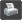Show Printable Version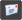Email this Page Display Modes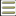Linear Mode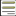Switch to Hybrid Mode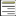Switch to Threaded Mode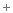Posting Rules You may not post new threads You may not post replies You may not post attachments You may not edit your posts vB code is On Smilies are On [IMG] code is On HTML code is Off